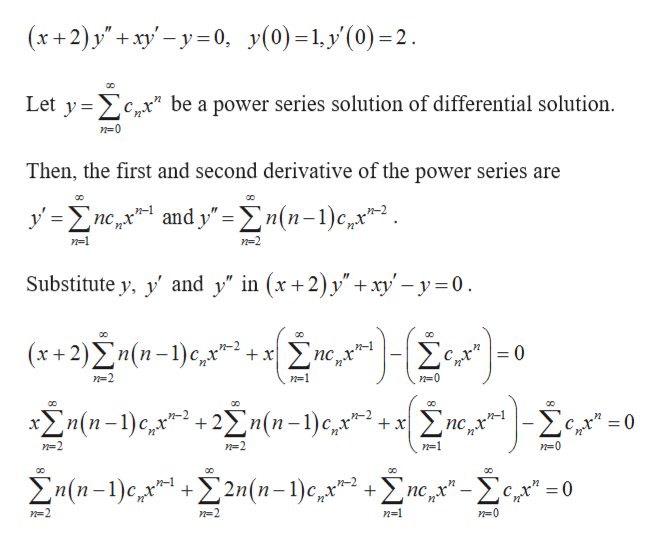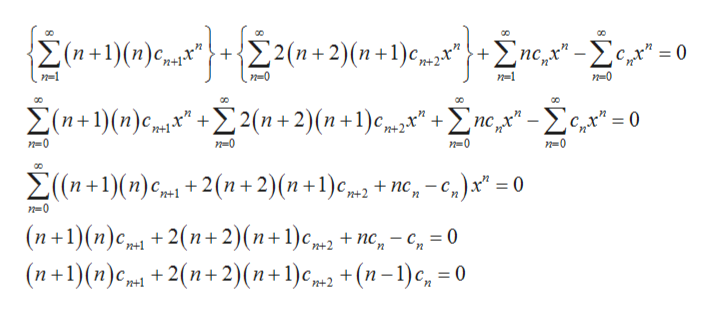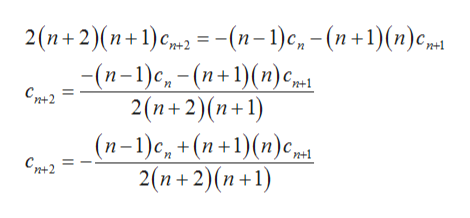Differential equationsFind the particular solution to the initial value problem:(x+2)y"+xy'-y=0,    y(0)=1, y'(0)=2

Question

Differential equations

Find the particular solution to the initial value problem:

(x+2)y"+xy'-y=0,    y(0)=1, y'(0)=2

Step 1

Consider the differential equation.help_outlineImage Transcriptionclose(x+2) y'+ xy-y0 y(0)1y(0)2 Let y c,*" be a power series solution of differential solution Then, the first and second derivative of the power series are yneandy=n(n-1)c2 ncx n-1 n-2 Substitute y, yand y" in (x+2) y" +xy'-y0 (x+2)2n(n-)e, n-1 =0 nc +x - n=2 n=1 n(n-)C2n(1-1)e,2 0 x x n=2 n=2 n=1 n=0 n(n-1)e 2n(n-1)c,x = 0 + n-2 n=2 n=1 n=0 fullscreen
Step 2

Shift n = n + 1 and n = n + 2 in the first and second summation, respectively.help_outlineImage Transcriptionclose{2 (n+1) (п)с. 22(n+2)(п+1) с+2 пс - Хс, -0 п-1 п-0 п-1 п-0 оо оо оо Х(n+1)(п)с 2 2(п + 2)(п +1) с " + Zпе,у" - Хсд" -0 п-0 n0 n0 n-0 ос 2 (n+1)(п)с, + 2(n+ 2)(n +1)сm, + nе,- 0 п0 (п+1)(п)ср, + 2(п+ 2) (п+1)с (п+1)(п)с + 2(п+ 2) (n+1)с, + (n-1)с, 30 + nc, - с, — 0 n+2 у fullscreen
Step 3

Isolate cn + 2.

...help_outlineImage Transcriptionclose2 (п+ 2)(п+1)с, —-(п-1) с, - (п+1)(п)срн (п-1)с, - (п+1)(п) с,ри 2(n+2)(п+1) (п-1)с, +(п+1)(n)с, Сд2 Сре2 2(п+2)(п+1) fullscreen

Want to see the full answer?

See Solution

Want to see this answer and more?

Our solutions are written by experts, many with advanced degrees, and available 24/7

See Solution
Tagged in

Math